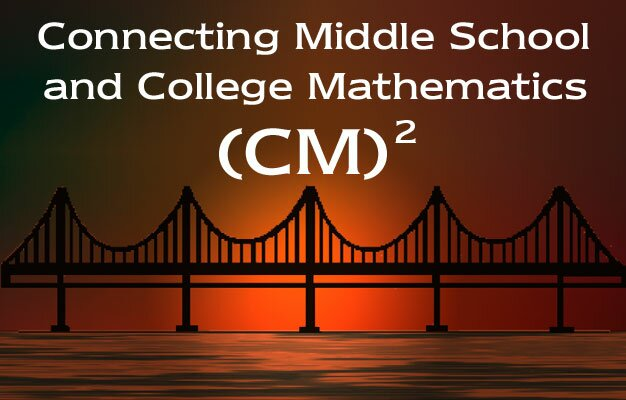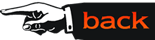### Courses

• Data Analysis and Probability: Course topics include: theoretical and experimental probabilities, simulation of random phenomena, probability of the union and intersection of two events, probability of the complement of an event, conditional probability including dependent and independent events, and geometric approaches to probability. Key elements of data analysis include: measures of central tendency and dispersion, correlation and regression, prediction and error, random variables, expected value of a random variable, and counting techniques including permutations and combinations. Graphing calculators and simulation software are utilized throughout the course. Prerequisite: College Algebra

• Algebraic Structures and Number Theory: Course topics include: a detailed study of the integers and the rational numbers with special emphasis on the division algorithm, Greatest Common Divisor (GCD) and Least Common Multiple (LCM), Euclids Algorithm, GCD Identity, prime numbers, The Fundamental Theorem of Arithmetic, Pythagorean triples, modular arithmetic, and natural generalizations to polynomials (with integer or rational coefficients) and other axiomatic structures. Graphing calculators, equipped with symbolic algebra capabilities, are used extensively throughout the course. Prerequisite: College Algebra

• Geometric Structures: Course topics include: concepts in Euclidean Geometry, similarity, transformations, coordinate geometry, vectors, complex numbers in geometric transformations, surface area, volume, and spherical geometry. The Geometers Sketchpad (GSP) are employed throughout the course. Prerequisite: College Algebra and elementary trigonometry

• Mathematics of Change (Calculus): Course topics include: slope, rates of change (differentiation), optimization, graphing, area (integration), and volume. An important aspect of the course is the use of calculus tools in solving real life problems in finance, business, economics, and other applied areas. Graphing calculators are creatively employed for exploration and computation. Prerequisite: College Algebra and elementary trigonometry